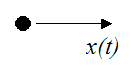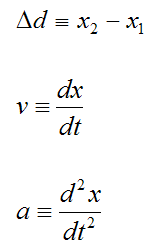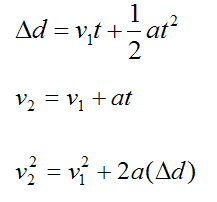# Motion Along A Straight Line

The following particle is moving in a straight line.Define the following variables:

x(t) is the position of the particle as a function of time

Δd is the change in position of the particle

v is the velocity of the particle

a is the acceleration of the particle

t is time

Now,If we assume constant acceleration, we have the following formulas. Note that the subscripts 1 and 2 denote "initial" and "final".These formulas also apply to bodies which are moving in a straight line, with no rotation. This means that every particle in the body is following the same path.